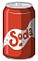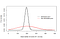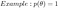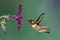# The Deal with the DenominatorA can of soda will have have some volume of soda to consume

The denominator of Bayes Rule is arguably the most important part of the formula.

# Motivation:

Why?

Let’s go through the formula piece by piece for a second:

# Sensitivity and Zero Priors

What do we mean by sensitivity?

A general rule of thumb is, when looking at Bayes Rule, the component (either likelihood or prior) with the most extreme LOWER value will affect the posterior the most.

Now,

It is also true that the MORE data we have, the LESS of an effect the prior has.

In contrast, the LESS data we have, the GREATER of an effect the prior has.

— — — — — — — — — — — — — — — — — — — — — — — — — — — — — — —

# Uninformative Priors, Informative Priors

Two more types of priors are uninformative and informative priors.An example of an uninformative and informative prior viewed simultaneously. (Image Credits: https://bookdown.org/mandyyao98/bookdown-demo-master/lecture-6-bayesian-inference-for-means.html)

# Uninformative Priors

Like we mentioned in the previous post on priors, all priors contain some information.

Although Uninformative priors are often used in attempt to produce an “objective analysis”, there is no such thing! So this kind of prior is used when there is an analysis at stake for being as objective as possible.

One pitfall of uninformative priors is that they can often be unbounded and therefore: not make practical sense and not be proper probability distributions.

An example of this kind of prior could be one that is for a…

# Prior and Likelihood Influence (a visualization)

Let’s use a Bayes Box to illustrate how priors and likelihoods affect the shape of the corresponding posterior distributions!

A Bayes Box is a table that walks you through the calculation of the posterior. There is a column for each part of the equation:

Let’s look back at our apple bobbing example from.

Let’s suppose we have a bucket with 4 apples. These apples can be either red or green.

Let’s use a Bernoulli likelihood where X = 0 means a red apple was caught, and X= 1 means a green apple was caught.

Let’s let our parameter theta be…

# Flat Priors

In the fashion where a mathematical proof introduces a base case as the first example, we will do this for priors as well.

A flat prior essentially demands that p(theta) is held at a constant value (usually a natural number).You can use any constant, but this prior that equals one is called the “unity prior”.

So what does having a constant value prior mean to us? It assumes that all parameter values are equally likely to appear in a set of samples from a population.

It causes the posterior to be essentially entirely affected by the likelihood alone. The posterior becomes some fraction of the likelihood. …

# Priors : Initial Beliefs

In this post, we will be introducing the likelihood’s neighbor:A wonderful version of Bayes Rule presented by Ben Lambert in his text A Student’s Guide to Bayesian Statistics

Priors. They are exactly what it sounds like. They represent our most current, basic understanding and interpretation of a phenomena.

Here, we present a few ways of understanding what a prior is. We will go through each one.

# Bruno de Finetti and Exchangeability

You may recall that likelihoods are constructed from the product of many individual likelihoods.

In order for us to claim this, we must ensure our sample we are basing our likelihoods from is independent and identically distributed or random.

However, this can be a tricky condition to meet.

Thanks to Italian probabilistic statistician Bruno de Finetti, Bayesian may use a similar condition, exchangeability (for large enough samples).

What does it mean for a sequence of random variables to be exchangeable?

You may be wondering, if a sequence is exchangeable, is the sequence of random variables equally likely?

This is where…

# Equivalence (Likelihoods)

There are many equivalence relations you might come across in your own mathematical journeys, but there is one particularly useful one acknowledged in Bayesian Statistics.

Before we take a look at it, let’s do a little thought exercise:

Suppose we have a probability of flipping heads for a coin , X.

Then, we can easily find the probability of getting 2 heads out of 2 flips, knowing that the probability of flipping heads is X.

A harder question to answer, however, is what is the likelihood that the probability of flipping heads is X, given only a dataset of 2…

# Little bit of Likelihoods: a more Analytical Approach

For those of you who would prefer a more mapped out relationship between probability distributions and likelihoods, please refer to the map below.

Here is an example using integrals to show that Likelihoods do not sum to 1 for all values of theta:

# Little bit of LikelihoodsThe number of times this hummingbird flaps its wings or number of seconds it feeds from the flower are observations. (Image Creds: Matt Cuda/Getty)

The beauty of Bayesian inference is that it holds all relevant observations as dependable truth, rather than viewing the output of nature’s whirring as unreliable.

From here on out, we will refer to these observations as “data”.

Now, when we want to model the process that underpins these data, Bayesian inference tells us that the data is fixed or unchanging, while the parameters than we use to model can be modified as necessary.

Likelihoods allows us to adjustable parameters, so that the most “ideal” set of parameters is used to model a desired process.

# Probability Distributions vs. Likelihoods

If you have taken a statistics…## fieldnotes

All proofs are mine, unless indicated.